# ggExtra - Add marginal histograms to ggplot2, and more ggplot2 enhancements

ggExtra is a collection of functions and layers to enhance ggplot2. The main function is ggMarginal, which can be used to add marginal histograms/boxplots/density plots to ggplot2 scatterplots. You can view a live interactive demo to see some of its functionality.

Most other functions/layers are quite simple but are useful because they are fairly common ggplot2 operations that are a bit verbose.

This is an instructional document, but I also wrote a blog post about the reasoning behind and development of this package.

Note: it was brought to my attention that several years ago there was a different package called ggExtra, by Baptiste (the author of gridExtra). That old ggExtra package was deleted in 2011 (two years before I even knew what R is!), and this package has nothing to do with the old one.

## Installation

ggExtra is available through both CRAN and GitHub.

To install the CRAN version:

install.packages("ggExtra")

To install the latest development version from GitHub:

install.packages("devtools")
devtools::install_github("daattali/ggExtra")

ggExtra comes with an addin for ggMarginal(), which lets you interactively add marginal plots to a scatter plot. To use it, simply highlight the code for a ggplot2 plot in your script, and select ggplot2 Marginal Plots from the RStudio Addins menu. Alternatively, you can call the addin directly by calling ggMarginalGadget(plot) with a ggplot2 plot.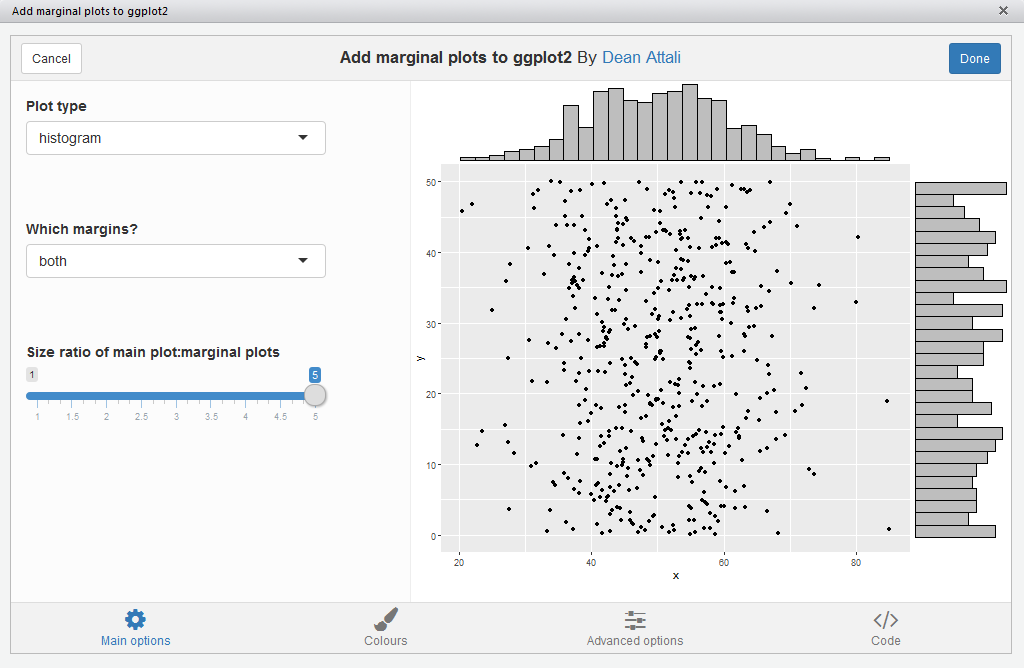## Usage

We’ll first load the package and ggplot2, and then see how all the functions work.

suppressPackageStartupMessages({
library("ggExtra")
library("ggplot2")
})

## ggMarginal - Add marginal histograms/boxplots/density plots to ggplot2 scatterplots

You need to have the grid and gtable packages installed for this function.

This function is meant to work as an easy drop-in solution for adding marginal density plots/histograms/boxplots to a ggplot2 scatterplot. You can either pass it a ready ggplot2 scatterplot and it will add the marginal plots, or you can just tell it what dataset and variables to use and it will generate the scatterplot plus the marginal plots.

As a simple first example, let’s create a dataset with 500 points where the x values are normally distributed and the y values are uniformly distributed, and plot a simple ggplot2 scatterplot.

set.seed(30)
df1 <- data.frame(x = rnorm(500, 50, 10), y = runif(500, 0, 50))
(p1 <- ggplot(df1, aes(x, y)) + geom_point() + theme_bw())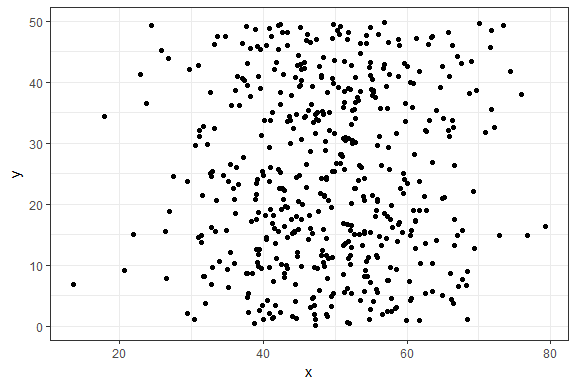Ok, now let’s add marginal density plots.

ggMarginal(p1)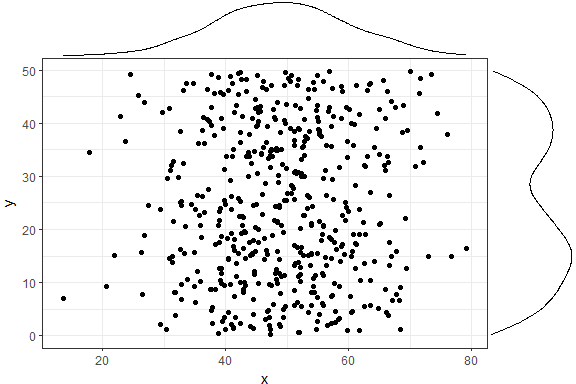That was easy. Notice how the syntax is not following the standard ggplot2 syntax - you don’t “add” a ggMarginal layer with p1 + ggMarginal(), but rather ggMarginal takes the object as an argument and returns a different object ggMarginal(p1). This means that you can use magrittr pipes, for example p1 %>% ggMarginal.

Let’s make the text a bit larger to make it easier to see.

ggMarginal(p1 + theme_bw(30) + ylab("Two\nlines"))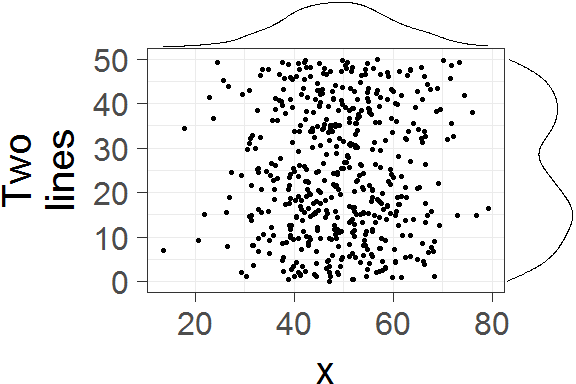Notice how the marginal plots occupy the correct space, and even when the main plot’s points are pushed to the right because of larger text or longer axis labels, the marginal plots automatically adjust.

You can also show histograms instead.

ggMarginal(p1, type = "histogram")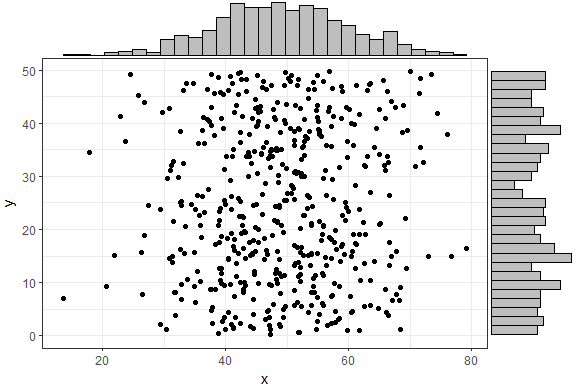There are several more parameters, here is an example with a few more being used. Note that you can use any parameters that the geom_XXX layers accept, and they will be passed to those layers, such as col and fill in the following example.

ggMarginal(p1, margins = "x", size = 2, type = "histogram",
col = "blue", fill = "orange")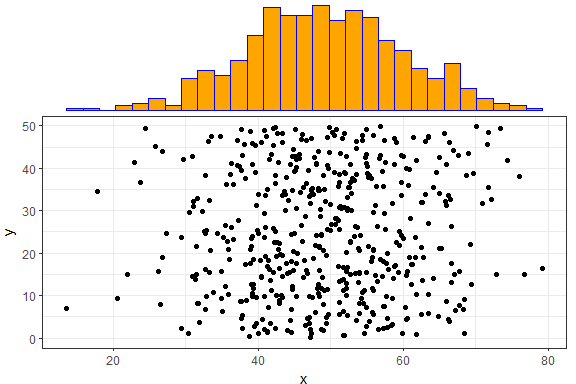In the above example, size = 2 means that the main scatterplot should occupy twice as much height/width as the margin plots (default is 5). The col and fill parameters are simply passed to the ggplot layer for both margin plots.

If you want to specify some parameter for only one of the marginal plots, you can use the xparams or yparams parameters, like this:

ggMarginal(p1, type = "histogram", xparams = list(binwidth = 1, fill = "orange"))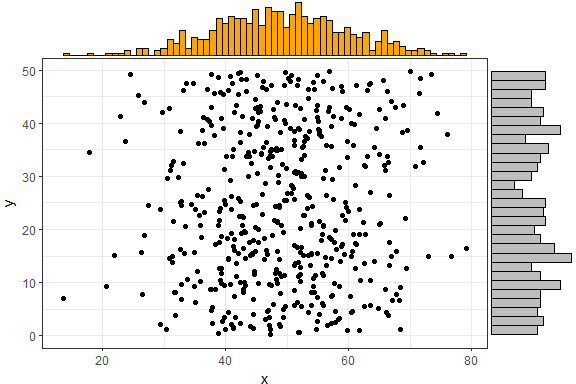You don’t have to supply a ggplot2 scatterplot, you can also just tell ggMarginal what dataset and variables to use, but of course this way you lose the ability to customize the main plot (change text/font/theme/etc).

ggMarginal(data = mtcars, x = "wt", y = "mpg")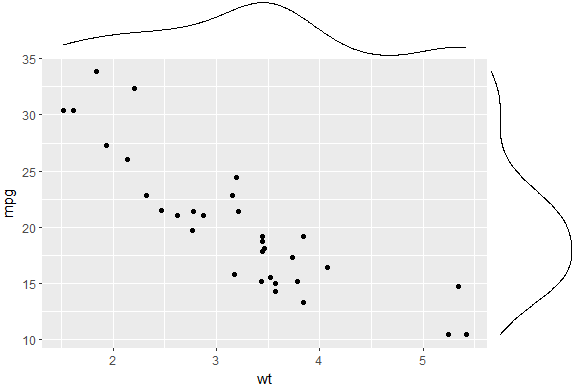Last but not least - you can also save the output from ggMarginal and display it later. (This may sound trivial, but it was not an easy problem to solve - see this discussion).

p <- ggMarginal(p1)
pFor more information, see ?ggExtra::ggMarginal.

## removeGrid - Remove grid lines from ggplot2

This is just a convenience function to save a bit of typing and memorization. Minor grid lines are always removed, and the major x or y grid lines can be removed as well (default is to remove both).

removeGridX is a shortcut for removeGrid(x = TRUE, y = FALSE), and removeGridY is similarly a shortcut for… .

df2 <- data.frame(x = 1:50, y = 1:50)
p2 <- ggplot2::ggplot(df2, ggplot2::aes(x, y)) + ggplot2::geom_point()
p2 + removeGrid()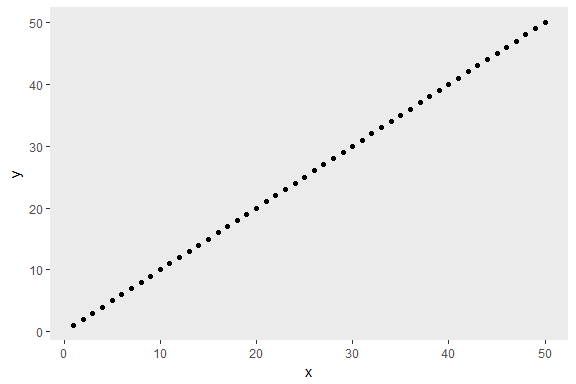For more information, see ?ggExtra::removeGrid.

## rotateTextX - Rotate x axis labels

Often times it is useful to rotate the x axis labels to be vertical if there are too many labels and they overlap. This function accomplishes that and ensures the labels are horizontally centered relative to the tick line.

df3 <- data.frame(x = paste("Letter", LETTERS, sep = "_"),
y = seq_along(LETTERS))
p3 <- ggplot2::ggplot(df3, ggplot2::aes(x, y)) + ggplot2::geom_point()
p3 + rotateTextX()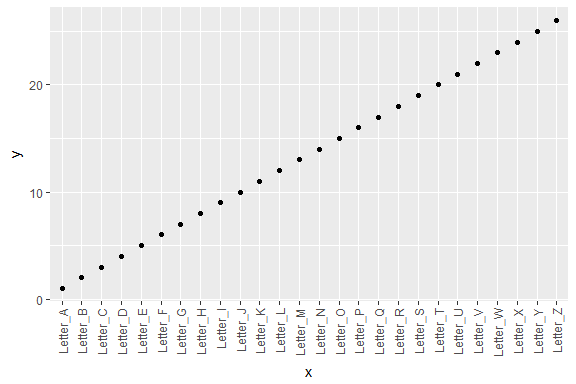For more information, see ?ggExtra::rotateTextX.

## plotCount - Plot count data with ggplot2

This is a convenience function to quickly plot a bar plot of count (frequency) data. The input must be either a frequency table (obtained with base::table) or a data.frame with 2 columns where the first column contains the values and the second column contains the counts.

An example using a table:

plotCount(table(infert\$education))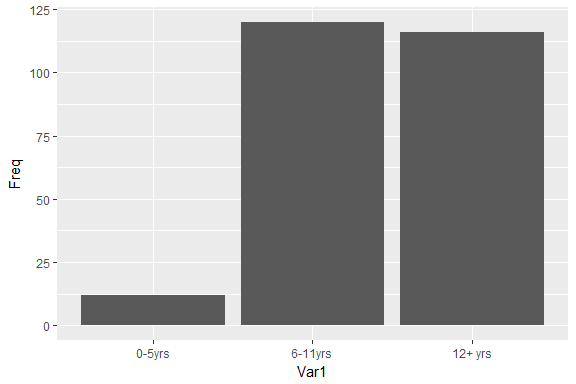An example using a data.frame:

df4 <- data.frame("vehicle" = c("bicycle", "car", "unicycle", "Boeing747"),
"NumWheels" = c(2, 4, 1, 16))
plotCount(df4) + removeGridX()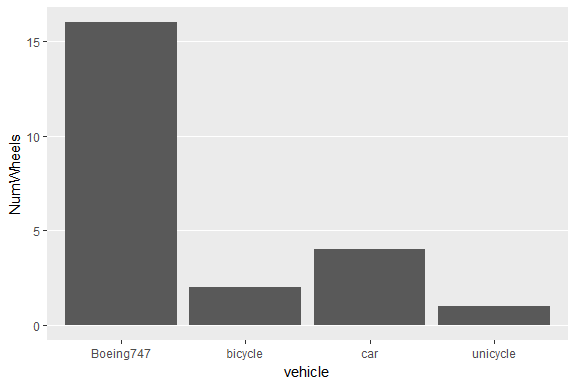For more information, see ?ggExtra::plotCount.